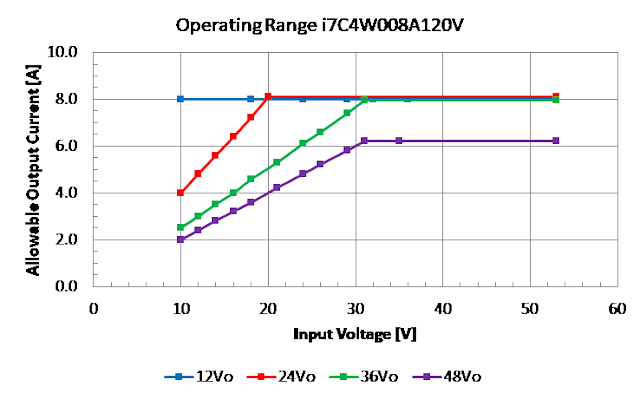Friday, August 28, 2020

Buck & Buck-Boost Converter Operating Ranges

This is a follow-on to a recent post where I discussed the difference between a buck, boost and buck-boost converter.  Now we will review the operating ranges of a buck and a buck-boost converter.

As a quick recap - buck and buck-boost DC-DC converters are widely used in power supply designs, and have been for many years.  They are popular because of their simplicity, low cost and high efficiency.  There is no transformer used in the design, and as such, there is no isolation between the input voltage and the output voltage.  Manufacturers like TDK-Lambda offer both buck and buck-boost converters.  With less components and complexity, a buck converter will offer a lower cost, higher efficiency and either a smaller package or more output power than an isolated converter.

As with any power supply and DC-DC converter, there are limits to their operation.  A programmable power supply like TDK-Lambda’s GH10-150 is rated to operate from 0 to 10V with a maximum current of 150A with a maximum output power of 1500W.  It is rather unreasonable to expect it to deliver 5V at 300A.

The main restriction of a non-isolated (step down) buck DC-DC converter is that the input voltage has to be higher than the output voltage. One example is the i6A4W010A033V-001-R which has an input range of 9 to 53Vdc, an output adjustment range of 3.3 to 40V and a maximum output current of 10A.  Figure 1 shows the relationship between the input voltage and the allowable output voltage.  Only the maximum output power restricts the amount of output current that can be produced.

Figure 1: i6A4W 10A buck converter output versus input voltage

A non-isolated buck boost (step-up / step-down) DC-DC converter has different constraints to its operation, besides the rated output power, current and voltage.  The TDK-Lambda i7C series has quite clear operating parameters on its datasheet, so we will use that as an example.

The part number i7C4W008A120V-001-R has an input voltage range of 9 to 53V, an output adjustment range of 9.6 to 48V and a maximum output current of 8A. In the datasheet the operating range is defined by two plots. These show the valid input and output voltage operating range and the maximum output current against input and output voltage.

Figure 2 is a pictorial representation of the valid input and output voltage operating range stated in the datasheet.  Note this differs significantly from the buck converter discussed previously, as the buck-boost topology allows it to generate voltages lower and higher than the input voltage.

Figure 2: i7C 8A valid input and output voltage operating range

From Figure 3, we can see the x axis matches the 9.6 to 53V input range stated on the datasheet and Figure 2.  The y axis has a maximum limit of 8A for the output current. There are four plots for different output voltages.Figure 3: i7C 8A Maximum output current vs the input and output voltage

As an example, a machine has 24Vdc available from an existing AC-DC DIN rail power supply. A new feature, which requires 48Vdc at 3A is required.  One way of generating this additional output voltage is to use a non-isolated DC-DC converter, like the i7C4W008A120V-001-R. We would use the purple plot (48Vo) and see that the maximum current we can safely draw from the converter is around 5A.  If the DIN rail power supply had a 24V battery back-up feature and the AC power failed, the i7C would continue to deliver 48V at 3A until the battery discharged down to 15V.

When a buck-boost DC-DC converter has a low input voltage, the input current switched by the FET is much larger than when it is operating at a high input voltage. This is the main restriction to the amount of output power it can deliver.

Like any power supply or DC-DC converter, having determined that the electrical parameters have been met, proper thermal management then has to be followed to ensure long term, reliable operation.

Figure 4: TDK-Lambda’s i7C 300W buck-boost DC-DC converter

Power Guy